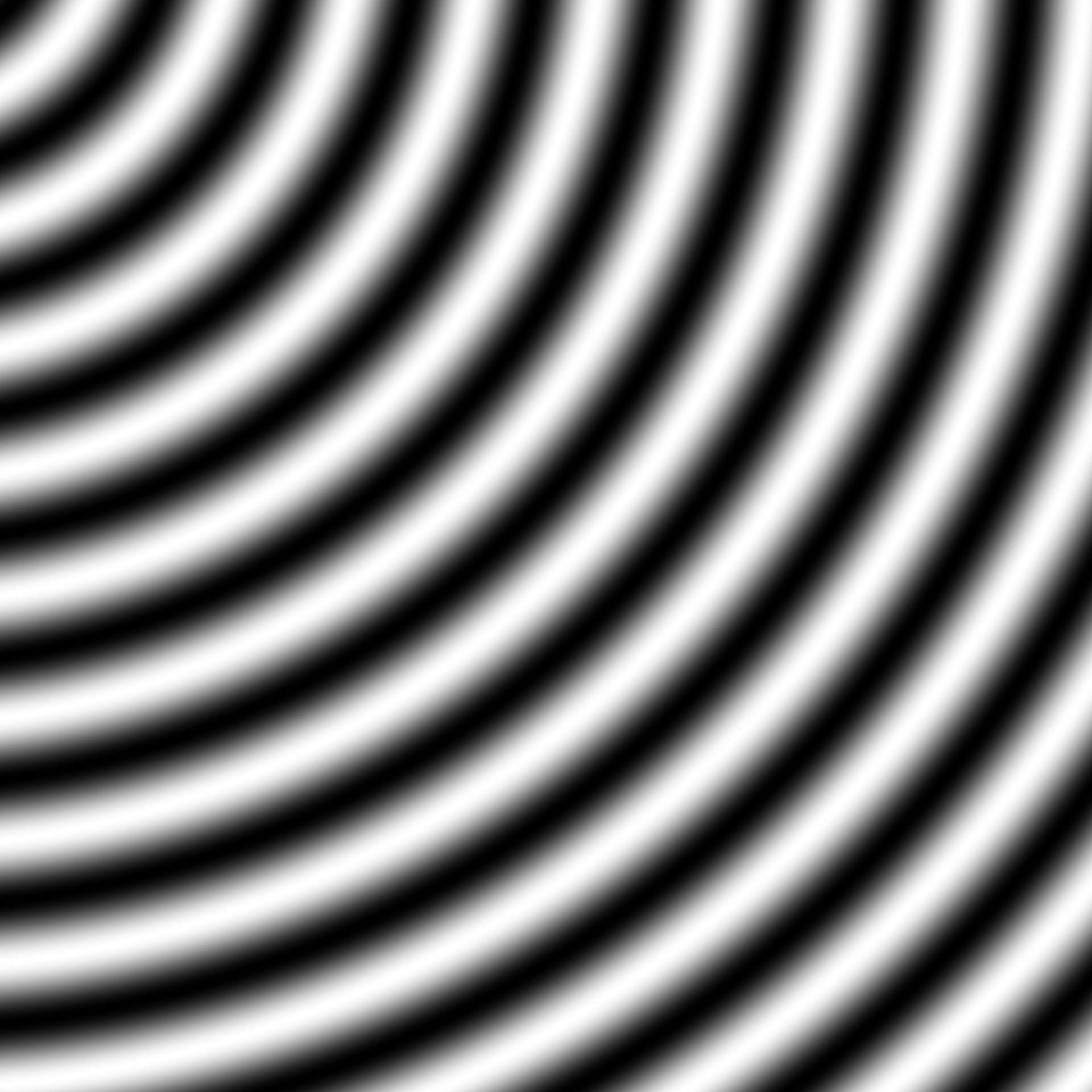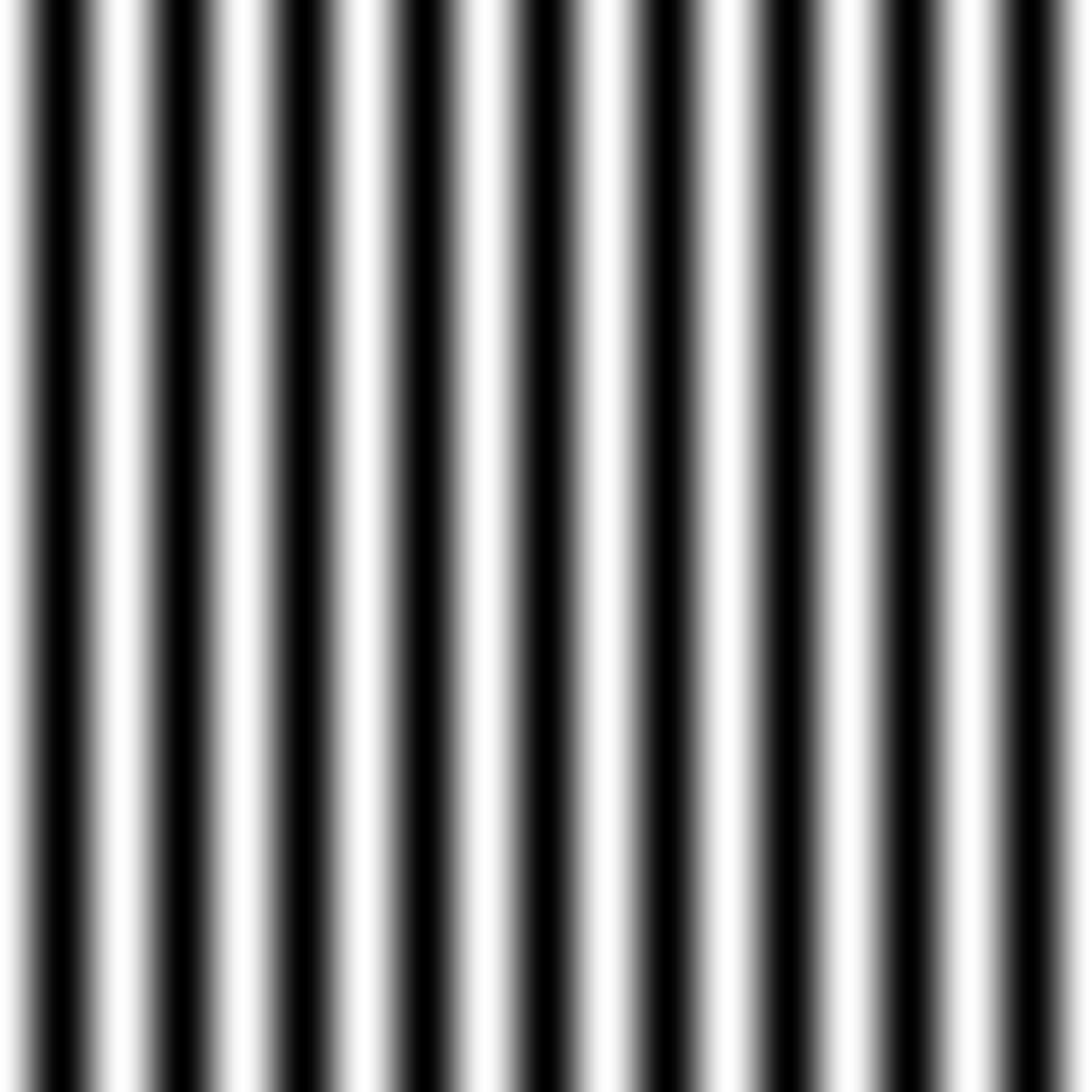This generator generates multidimensional waves based on cos to the distance to the center. This means that you can create ripple waves or parallel waves depending on how many dimensions you provide. The output is scaled between -1 and 1 and the frequency determines the number of waves per unit. The result is much like gen_spheres() but has smooth transitions at each extreme.

gen_waves(x, y = NULL, z = NULL, t = NULL, frequency = 1, ...)

## Arguments

x, y, z, t The coordinates to get pattern from The frequency of the generator ignored

## Value

A numeric vector

Other Pattern generators: gen_checkerboard(), gen_spheres()

## Examples

grid <- long_grid(seq(1, 10, length.out = 1000), seq(1, 10, length.out = 1000))
grid$ripple <- gen_waves(grid$x, grid$y) grid$wave <- gen_waves(grid\$x)

plot(grid, ripple)plot(grid, wave)